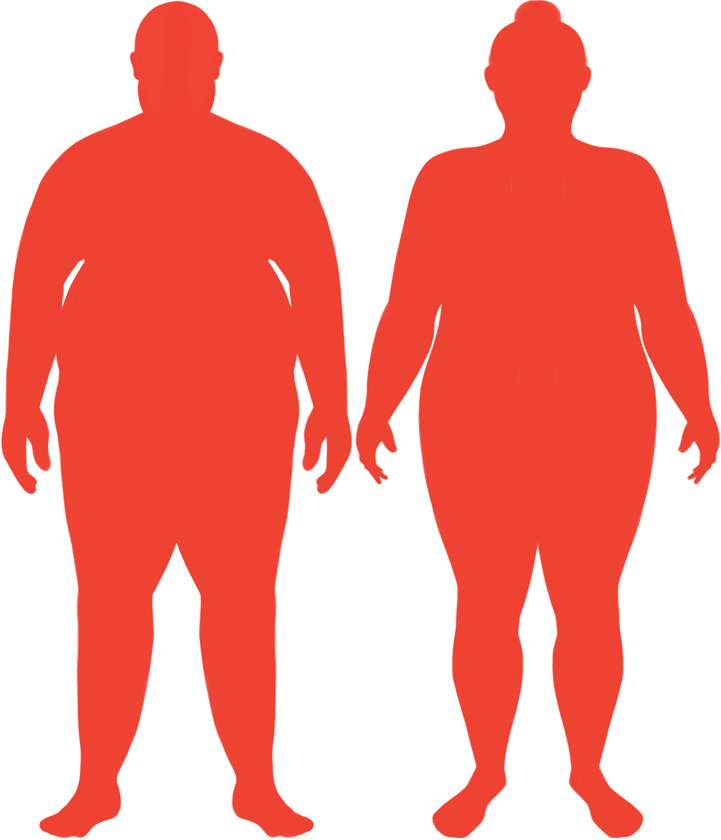# BMI Calculator

Result for 5'1" and 283 lbs.
Change Here.53.47

### Obese BMI

At 5'1" and 283 Pounds your BMI is 53.47

### BMI Scale

 Under Normal Over Obese
-124 lbs
to reach an
overweight bmi classification.
##### Overweight BMI Ends:
158.8 lbs
-151 lbs
to reach a
normal bmi classification.
132.3 lbs

### BMI Grading Table2 for Height 5'1"

 Weight Weight Class Below 97.9 lbs Underweight 97.9 lbs - 132.3 lbs Normal Weight 132.3 lbs - 158.8 lbs Overweight 158.8 lbs and Above Obese

### Weight Loss

If you were seeking a normal BMI weight of 132.3lbs, and you lost two pounds a week4, then you could reach a normal BMI in
76 Weeks
Or to be considered overweight, a goal weight of 158.8 lbs could be reached in
62 Weeks

### Obese BMI Silhouette### Ideal Weight for a 5 foot 1 Male or Female2

The ideal weight range using the BMI for a male or female with a height of 5'1" is

### Between

97.9 lbs

and
132.3 lbs
How to Calculate BMI3 for 5'1" and 283 lbs
(5 x 12) + 1 = 61
(283 / (61 x 61)) x 703
= 53.466541252352
5 = foot | 1 = inch | 283 = weight lbs

### Conversion

• 5 foot 1 = 61 inches
• 5 foot 1 = 154.94cm
• 283 pounds = 20 stone 3 pounds
• 283 pounds = 128.37 kg
• 283 pounds = 128366.64 grams

### 5'1" and 283 lbs Summary

What is the BMI for a 5'1" and 283 lbs female? 53.47 BMI, Obese.
What is the BMI for a 5'1" and 283 lbs male? 53.47 BMI, Obese.
What is the ideal weight for a 5'1" female? Between: 97.9lbs and 132.3lbs
What is the ideal weight for a 5'1" male? Between: 97.9lbs and 132.3lbs
If I am 5ft 1in and weigh 283 lbs, is that a good weight for my height? Under the BMI classification, 283 lbs is classed as being Obese.